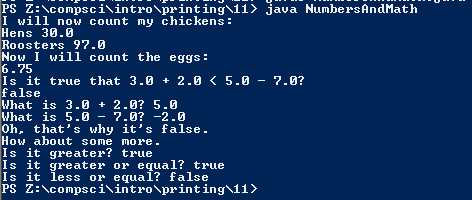# Assignment #11

## Code

```    /// Name: Kabir Virk
/// Period: 6
/// Program Name: NumbersAndMath.java
/// File Name: NumbersAndMath
/// Date Finished: 9/14/2015
public class NumbersAndMath
{
public static void main( String[] args )
{
//this line is printing the words
System.out.println( "I will now count my chickens:" );
//these lines prints the words and solves the math problem
System.out.println( "Hens " + ( 25.0 + 30.0 / 6.0 ) );
System.out.println( "Roosters " + ( 100.0 - 25.0 * 3.0 % 4.0 ) );
//this line prints the type
System.out.println( "Now I will count the eggs:" );
//this line is printing the type
System.out.println( 3.0 + 2.0 + 1.0 - 5.0 + 4.0 % 2.0 - 1.0 / 4.0 + 6.0 );
//this line is solving a math problem
System.out.println( "Is it true that 3.0 + 2.0 < 5.0 - 7.0?" );
//these lines print the type and solve a math problem
System.out.println( 3.0 + 2.0 < 5.0 - 7.0 );
//this line prints the type
System.out.println( "What is 3.0 + 2.0? " + ( 3.0 + 2.0 ) );
System.out.println( "What is 5.0 - 7.0? " + ( 5.0 - 7.0 ) );
//this line prints the type
System.out.println( "Oh, that's why it's false." );
//this line prints the type
System.out.println( "How about some more." );
//these next three lines print the words and solve the math problems
System.out.println( "Is it greater? " + ( 5.0 > -2.0 ) );
System.out.println( "Is it greater or equal? " + ( 5.0 >= -2.0 ) );
System.out.println( "Is it less or equal? " + ( 5.0 <= -2.0 ) );
}
}
```

### Picture of the output